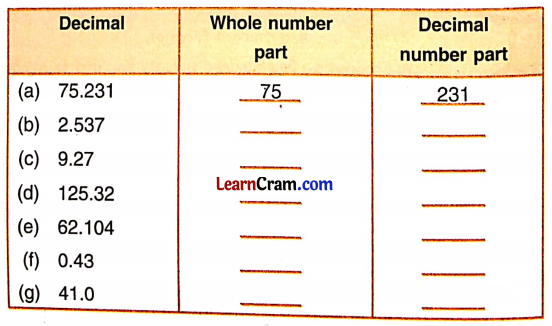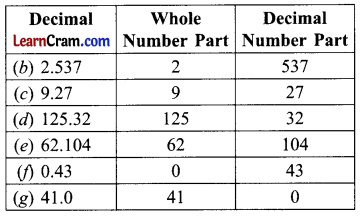# DAV Class 5 Maths Chapter 5 Worksheet 2 Solutions

The DAV Class 5 Maths Book Solutions Pdf and DAV Class 5 Maths Chapter 5 Worksheet 2 Solutions of Decimals offer comprehensive answers to textbook questions.

## DAV Class 5 Maths Ch 5 Worksheet 2 Solutions

Question 1.
(a) 5.3
Solution:
Five point three

(b) 71.8
Solution:
Seventy one point eight

(c) 182.135
Solution:
One hundred eighty two point one three five

(d) 172.001
Solution:
One hundred seventy two point zero zero one(e) 1.839
Solution:
One point eight three nine

(f) 91.99
Solution:
Ninety one point nine nine

(g) 2001.201
Solution:
Two thousand one point two zero one

(h) 100.100
Solution:
Hundred point one

Question 2.
Write the number names. The first one is done for you.
(a) 63.85
Solution:
Sixty three and eighty five hundredths

(b) 15.72
Solution:
Fifteen and seventy two hundredths

(c) 11.07
Solution:
Eleven and seven hundredths

(d) 9.24
Solution:
Nine and twenty four hundredths(e) 45.05
Solution:
Forty five and five hundredths

(f) 987.62
Solution:
Nine hundred eighty seven and sixty two hundredths

(g) 125.09
Solution:
One hundred twenty five and nine hundredths

(h) 100.97
Solution:
One hundred and ninety seven hundredths

(i) 8257.85
Solution:
Eight thousand two hundred fifty seven and eighty five hundredths

(j) 9.782
Solution:
Nine and seven hundred eighty two thousandths

(k) 15.674
Solution:
Fifteen and six hundred seventy four thousandths

(l) 67.005
Solution:
Sixty seven and five thousandths(m) 768.135
Seven hundred sixty eight and one hundred thirty five thousandths

Question 3.
Write in decimal form. The first one is done for you.
(a) Fifty seven and seven hundredths. _________
Solution:
57.07

(b) Sixty and one hundredths. _________
Solution:
60.01

(c) Twenty four and twelve hundredths. _________
Solution:
24.12

(d) Seventy six and five hundredths. _________
Solution:
76.05

(e) Two and four hundred seventy six thousandths. _________
Solution:
2.476

(f) Four and sixty seven hundredths. _________
Solution:
4.67

(g) Thirty two and fifty four thousandths. _________
Solution:
32.054(h) Five thousand six hundred seventy two and four hundred seventy eight thousandths. _________
Solution:
5672.478

Question 4.
Write the whole part and decimal number part in each of the following:Solution:Question 5.
Write the decimals in words. The first one is done for you.
(a) 468.103
Solution:
Four hundred sixty eight point one zero three.

(b) 7.8
Solution:
Seven point eight.

(c) 39.3
Solution:
Thirty nine point three.

(d) 65.92
Solution:
Sixty five point nine two.(e) 352.184
Solution:
Three hundred fifty two point one eight four.

(f) 647.003
Solution:
Six hundred forty seven point zero zero three.

(g) 1482.309
Solution:
One thousand four hundred eighty two point three zero nine.

(h) 1.943
Solution:
One point nine four three.

(i) 81.88
Solution:
Eighty one point eight eight.

Question 6.
Show each of the following decimals on the place value chart.
(a) 8.4
(b) 19.34
(c) 0.9921
(d) 9.009
(e) 3.333
(f) 0.075
(g) 5.7679
(h) 182.95
Solution:Question 7.
Write as decimals:
(a) 15$$\frac{3}{10}$$
Solution:
15.3

(b) 7$$\frac{8}{100}$$
Solution:
7.08

(c) 101$$\frac{12}{100}$$
Solution:
101.12

(d) 9$$\frac{153}{1000}$$
Solution:
9.153(e) 11$$\frac{11}{100}$$
Solution:
11.11

(f) 46$$\frac{1}{10}$$
Solution:
46.1

(g) 76$$\frac{2}{1000}$$
Solution:
76.002

(h) 5$$\frac{55}{100}$$
Solution:
5.55

DAV Class 5 Maths Chapter 5 Worksheet 2 Notes

Hundredth and Thousandth
Let us study the place value of 7 in these numbers.If 7 moves one more column to the right its place value becomes
7 ÷ 100 = $$\frac{7}{100}$$ = 7 hundredths or 0.07Fractions with denominators 10, 100, and 1,000 are called decimal fractions.
Example: Decimal part and whole number part
A decimal number consists of two parts

• Whole Number part
• Decimal Number part

These two parts are separated by a dot.While reading a decimal number, the whole number part is read as a whole, and the decimal number part is in separate digits.
Let us read some decimal numbers
4.5 = Four point five
81.36 = Eighty one point three six
786.008 = Seven hundred eighty six points zero zero eight.
175$$\frac{76}{100}$$ is called mixed fraction. We read it as one hundred seventy five and seventy six hundredths.